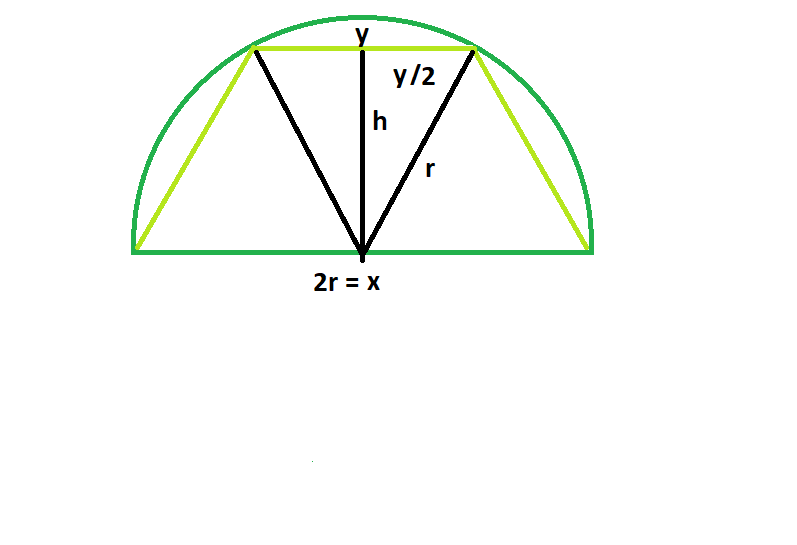# Largest trapezoid that can be inscribed in a semicircle

Given a semicircle of radius r, the task is to find the largest trapezoid that can be inscribed in the semicircle, with base lying on the diameter.

Examples:

```Input: r = 5
Output: 32.476

Input: r = 8
Output: 83.1384
```

## Recommended: Please try your approach on {IDE} first, before moving on to the solution.Approach: Let r be the radius of the semicircle, x be the lower edge of the trapezoid, and y the upper edge, & h be the height of the trapezoid.
Now from the figure,

r^2 = h^2 + (y/2)^2

or, 4r^2 = 4h^2 + y^2

y^2 = 4r^2 – 4h^2

y = 2√(r^2 – h^2)

We know, Area of Trapezoid, A = (x + y)*h/2

So, A = hr + h√(r^2 – h^2)

taking the derivative of this area function with respect to h, (noting that r is a constant since we are given the semicircle of radius r to start with)

dA/dh = r + √(r^2 – h^2) – h^2/√(r^2 – h^2)

To find the critical points we set the derivative equal to zero and solve for h, we get

h = √3/2 * r

So, x = 2 * r & y = r

So, A = (3 * √3 * r^2)/4

Below is the implementation of above approach:

## C++

 `// C++ Program to find the biggest trapezoid ` `// which can be inscribed within the semicircle ` `#include ` `using` `namespace` `std; ` ` `  `// Function to find the area ` `// of the biggest trapezoid ` `float` `trapezoidarea(``float` `r) ` `{ ` ` `  `    ``// the radius cannot be negative ` `    ``if` `(r < 0) ` `        ``return` `-1; ` ` `  `    ``// area of the trapezoid ` `    ``float` `a = (3 * ``sqrt``(3) * ``pow``(r, 2)) / 4; ` ` `  `    ``return` `a; ` `} ` ` `  `// Driver code ` `int` `main() ` `{ ` `    ``float` `r = 5; ` `    ``cout << trapezoidarea(r) << endl; ` `    ``return` `0; ` `} `

## Java

 `// Java Program to find the biggest trapezoid ` `// which can be inscribed within the semicircle ` ` `  `import` `java.util.*; ` `import` `java.lang.*; ` `import` `java.io.*; ` ` `  `class` `GFG{ ` `// Function to find the area ` `// of the biggest trapezoid ` `static` `float` `trapezoidarea(``float` `r) ` `{ ` ` `  `    ``// the radius cannot be negative ` `    ``if` `(r < ``0``) ` `        ``return` `-``1``; ` ` `  `    ``// area of the trapezoid ` `    ``float` `a = (``3` `* (``float``)Math.sqrt(``3``)  ` `            ``* (``float``)Math.pow(r, ``2``)) / ``4``; ` ` `  `    ``return` `a; ` `} ` ` `  `// Driver code ` `public` `static` `void` `main(String args[]) ` `{ ` `    ``float` `r = ``5``; ` `    ``System.out.printf(``"%.3f"``,trapezoidarea(r)); ` `} ` `} `

## Python 3

 `# Python 3 Program to find the biggest trapezoid  ` `# which can be inscribed within the semicircle  ` ` `  `# from math import everything ` `from` `math ``import` `*` ` `  `# Function to find the area  ` `# of the biggest trapezoid  ` `def` `trapezoidarea(r) : ` ` `  `    ``# the radius cannot be negative  ` `    ``if` `r < ``0` `: ` `        ``return` `-``1` ` `  `    ``# area of the trapezoid ` `    ``a ``=` `(``3` `*` `sqrt(``3``) ``*` `pow``(r,``2``)) ``/` `4` ` `  `    ``return` `a ` ` `  ` `  `# Driver code      ` `if` `__name__ ``=``=` `"__main__"` `: ` ` `  `    ``r ``=` `5` ` `  `    ``print``(``round``(trapezoidarea(r),``3``)) ` ` `  ` `  `# This code is contributed by ANKITRAI1 `

## C#

 `// C# Program to find the biggest  ` `// trapezoid which can be inscribed  ` `// within the semicircle ` `using` `System; ` ` `  `class` `GFG ` `{ ` `// Function to find the area ` `// of the biggest trapezoid ` `static` `float` `trapezoidarea(``float` `r) ` `{ ` ` `  `    ``// the radius cannot be negative ` `    ``if` `(r < 0) ` `        ``return` `-1; ` ` `  `    ``// area of the trapezoid ` `    ``float` `a = (3 * (``float``)Math.Sqrt(3) *  ` `                   ``(``float``)Math.Pow(r, 2)) / 4; ` ` `  `    ``return` `a; ` `} ` ` `  `// Driver code ` `public` `static` `void` `Main() ` `{ ` `    ``float` `r = 5; ` `    ``Console.WriteLine(``""` `+ trapezoidarea(r)); ` `} ` `} ` ` `  `// This code is contributed  ` `// by inder_verma `

## PHP

 ` `

Output:

```32.476
```

Attention reader! Don’t stop learning now. Get hold of all the important DSA concepts with the DSA Self Paced Course at a student-friendly price and become industry ready.

My Personal Notes arrow_drop_upCheck out this Author's contributed articles.

If you like GeeksforGeeks and would like to contribute, you can also write an article using contribute.geeksforgeeks.org or mail your article to contribute@geeksforgeeks.org. See your article appearing on the GeeksforGeeks main page and help other Geeks.

Please Improve this article if you find anything incorrect by clicking on the "Improve Article" button below.

Article Tags :
Practice Tags :

Be the First to upvote.

Please write to us at contribute@geeksforgeeks.org to report any issue with the above content.